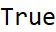# Wolfram CloudResource Object

Function Resource

# StrongPrimeQ

Determines if the given number is a strong prime, or not

 ResourceFunction["StrongPrimeQ"][p] gives True if p is a strong prime and False otherwise.

## Details and Options

StrongPrimeQ uses the number theoretic definition of a strong prime, not the cryptographic one.
StrongPrimeQ returns True if the given prime is greater than the arithmetic average of its nearest prime above and below it.

## Examples

### Basic Examples

The prime number 457 is strong:

 In:=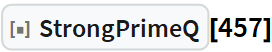Out=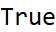It is bigger than the average of its preceding and succeeding primes:

 In:=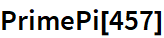Out=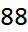In:=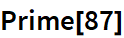Out=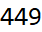In:=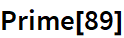Out=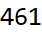In:=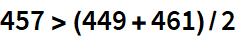Out=